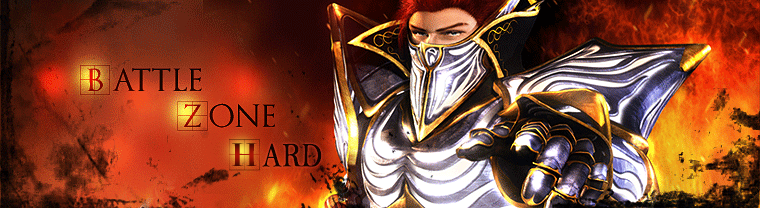Hello 172.70.34.57NewsRegisterDownloadsRankingswebshopvoteStatisticsBannedForumTop 10Top Guilds
 ID Name Score 1. A_Z_E 0``` `````` var montharray=new Array("Jan","Feb","Mar","Apr","May","Jun","Jul","A ug","Sept","Oc","Novem","Dec") function countup(yr,m,d){ var today=new Date() var todayy=today.getYear() if (todayy < 1000) todayy+=1900 var todaym=today.getMonth() var todayd=today.getDate() var todaystring=montharray[todaym]+" "+todayd+", "+todayy var paststring=montharray[m-1]+" "+d+", "+yr var difference=(Math.round((Date.parse(todaystring)-Date.parse(paststring))/(24*60*60*1000))*1) difference+=" days" document.write("Server Is Online From "+difference+" ") } countup(2009,12,28)  Blood Castle var minute2 = 55;var secundes2 = 38;bcin = minute2 * 60 + secundes2; if(minute2 > 30) doin = 3600 - bcin; else doin = -bcin; dobc = doin + 25*60; function changeeventtime2() { dobc = dobc - 1; if(dobc < -5*60) { dobc = 3600 + 30*60; dobc = dobc-1; } min2 = Math.floor(dobc/60); sec2 = dobc%60; if(min2 < 0) { document.getElementById("timespan2").innerHTML="Open"; } else { if(min2 < 10) min2 = "0"+min2; if(sec2 < 10) sec2 = "0"+sec2; document.getElementById("timespan2").innerHTML=min2+":"+sec2; } window.setTimeout("changeeventtime2()",1000); } changeeventtime2();  Devil Square var dods; var hour = 11;var minute = 55;var secundes = 38;dsin = minute * 60 + secundes; function changeeventtime() { dsin = dsin + 1; if(hour == 2 || hour == 4 || hour == 6 || hour == 8 || hour == 10 || hour == 12) { dods = 56*60 - dsin; } else { dods = 116*60 - dsin; } min = Math.floor(dods/60); sec = dods%60; if(dods < 0) { document.getElementById("timespan").innerHTML="Open"; } else { if(min < 10) min = "0"+min; if(sec < 10) sec = "0"+sec; document.getElementById("timespan").innerHTML=min+":"+sec; } window.setTimeout("changeeventtime()",1000); } changeeventtime(); ```